WEBINAR
Efficient Green Energy: Exploring Simulation-Driven Design for High-Torque Wind Turbine Machine
Thursday, September 21, 2023
Time
SESSION 1
SESSION 2
CEST (GMT +2)
03:00 PM
08:00 PM
EDT (GMT -4)
09:00 AM
02:00 PM
HOME / Applications / Electromagnetic Simulation of Brushless DC motor inside SOLIDWORKS

# Electromagnetic Simulation of Brushless DC motor inside SOLIDWORKS

## CAD modeling and simulation of BLDC motor

In this article, a BLDC motor has been created and simulated using respectively SOLIDWORKS and EMWorks2D (see Figure 1). The proposed model has been studied in . The BLDC model is automatically generated using MotorWizard. It is a motor software provided by EMWorks allowing both automatic design and simulation of electric motors. In addition to MotorWizard, EMWorks provides a 2D electromagnetic software (EMWorks2D) utilizing finite element method (FEM) and integrated inside SOLIDWORKS.

FEM simulation has proved its high capability to analyze complex electromagnetic problems and geometries . It has been applied to electrical machines since 1970s. Finite element analysis (FEA) helps to overcome the challenges faced in studying and designing electric machines by providing the ability to consider machine geometry, nonlinear behavior of the magnetic material and winding distribution in the model. Moreover, ability to apply different boundary conditions to the model and calculation of different types of losses, makes FEA useful solution.

Although 3D solution can ensure complete and accurate results by considering winding end effects, skewing effects, local effects, etc, - which is not the case in 2D simulation- it requires larger computation time and higher computer resources. Therefore, in design process or fault study of the machine where several models and conditions are studied, using 2D approach is more desirable due to lower computation time and acceptable results. This can help to accelerate the development of more efficient and innovative motors.

Figure 1 contains the 2D geometry of simulated BLDC motor. It consists of a 3-phase machine with 12 slots and 6 poles. Hence, the symmetry angle of this motor ismechanical degrees. The stator and rotor cores are made of steel (). The rotor poles, having surface mounted structure, are made of ferrite permanent magnets (). The shaft has non-magnetic material.

The double layers windings are arranged on 3 phases with a coil pitch equal to 1. Each phase has 1456 turns.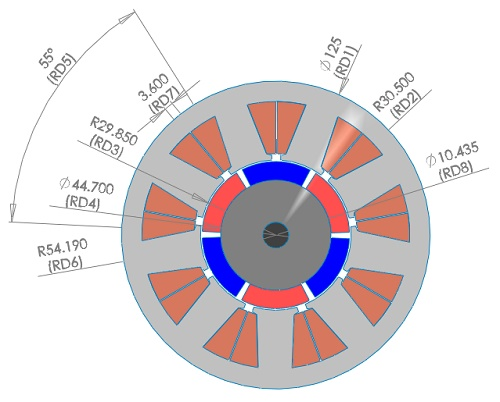Figure 1 - 2D model of the BLDC motor at the its initial position

Below is shown the excitation signal of each phase. It consists of 3 ideal square-waves currents. The three-phase winding is connected in wye. However, at each position only two phases are supplied, and one is not excited.  Switching on and off between the phases depends on the rotor position and is repeated every 360 electrical degrees of the rotation.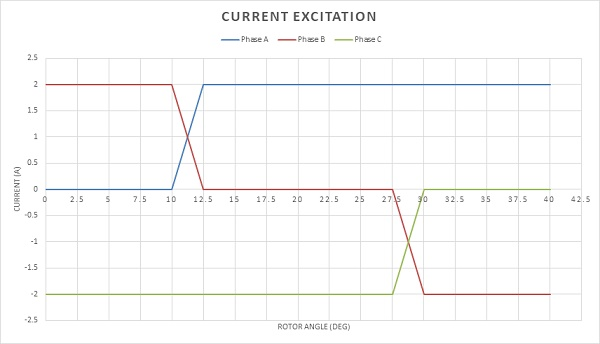Figure 2 - Excitation currents

Since Eddy effects and iron losses are neglected in this simulation, a static magnetic analysis is performed. A parametric sweep study, of both currents and rotor position, is defined to compute the output torque and magnetic flux results. Moreover, Inductance and flux linkage results will be generated. Torque results will be compared to ref  results.

## Meshing step

Meshing process is very crucial of an FEM simulation. Smaller elements size i.e. higher number of mesh elements, can enhance the results accuracy, but it may lead to increase the computation time. EMWorks2D comes with a flexible and user friendly mesher. It allows to add mesh control on specific surfaces and edges where the results could be very sensitive to the mesh. Figure 3 shows the meshed model while a mesh control is applied to the air gap.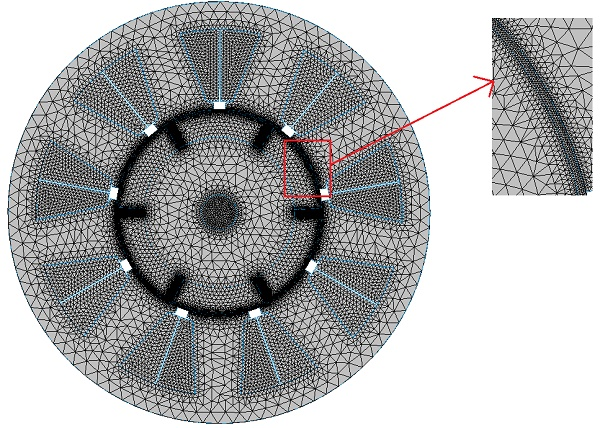Figure 3 - Meshed model

## Results and discussions

Simulation is run at different rotor position and results are generated. Figure 4a) and 4b) show the magnetic flux density respectively fringe and vector plots inside the machine at the initial position (0 deg, coil A not excited). The magnetic flux density is going from/to the machine center and this is due to the opposite polarity of phase B and C excited. High magnetic flux density can be observed in the stator teeth corners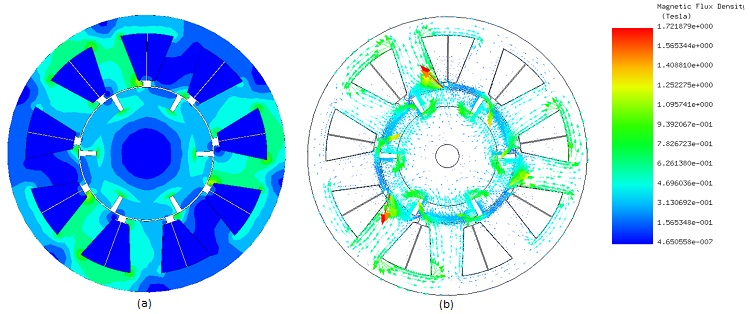Figure 4 - Magnetic flux density, a) fringe plot, b) vector plot

Figure 5 illustrates an animation plot of the magnetic flux lines versus rotor angle. Contour lines of the magnetic vector potential are plotted in Figure 6. It shows that almost of the magnetic field is circulating inside the motor.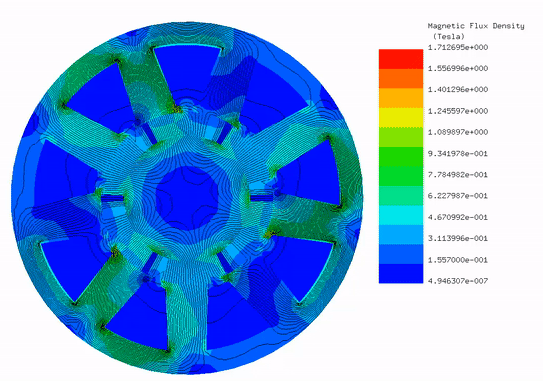Figure 5 - Animation of the magnetic flux density lines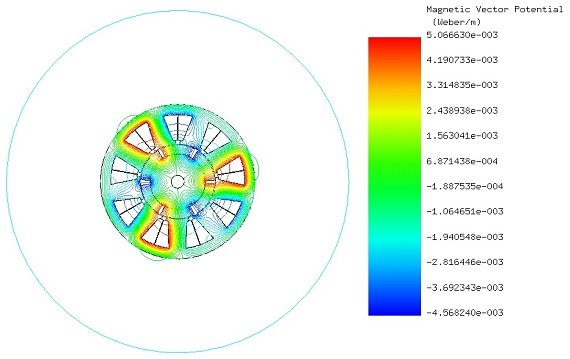Figure 6 - Contours line plot of the magnetic vector potential

Figure 7 contains an animation of the applied current density at different rotor position. In other words, Figure 7 shows the excitation of three phases at different rotor positions. This commutation allows developing the highest possible torque and reducing the torque ripple.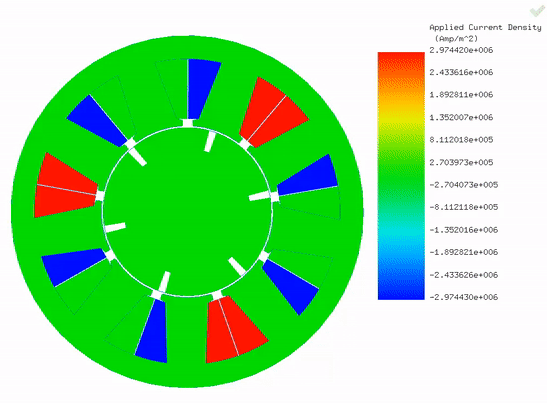Figure 7 - Animation of the applied current density

Figure below shows the load torque results computed by EMWorks2D for an angle range fromtoand compare them with the presented results in . The torque curve is characterized by small ripples amplitude but considerably a high number of ripples (totally 18 ripples in one full revolution). The maximum and the minimum values of the computed torque are respectively 2.05 N.m and 1.53 Nm. The average developed torque is around 1.89 Nm.

Figure 9 gives the static torque curve (average produced torque) versus ampere-turns. It shows that the torque is proportional to the ampere-turns. When there is no excitation, the torque value is around 0.1Nm (Cogging torque) and it increases to reach an average of 1.89 Nm at 2916 A-t. This linear dependency shown in the Figure 9 of the static torque generated by the BLDC is considerate as an advantage of brushed DC motor. Hence, it is an inherited advantage to the BLDC without using commutators and brushes.

Table 1 summarizes the inductance results of the motor phases. All the phases have the same inductances values. The flux linkage results of the three-phase windings are plotted in Figure 10.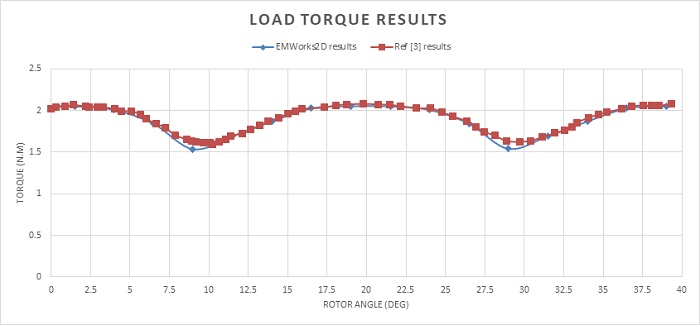Figure 8 - Torque curve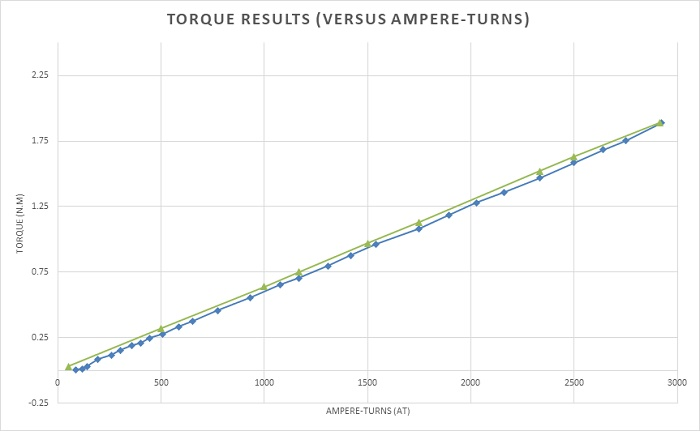Figure 9 - Torque behavior versus ampere-turns

Table 1 - Inductances results
 Phase A, B and C Self-inductance (H) 0.64085 Mutual inductance (H) 0.61795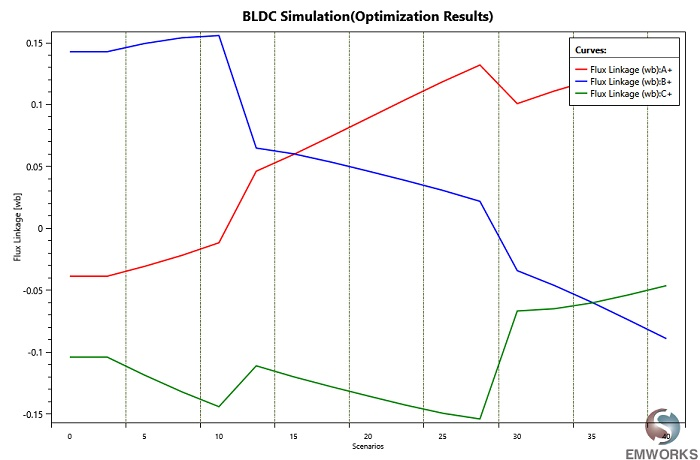Figure 10 - Flux linkage results

## Conclusion

The proposed BLDC motor was simulated using EMWorks2D. Magnetic flux, torque and inductance results are accurately generated versus rotor angle. 2D FEM solution provided by EMWorks2D helped to study efficiently the proposed model. A reduced computation time is achieved, and this can offer more simulation scenarios. However, 2D simulation has some drawbacks such as neglecting end effect, axial flux, skew which cause results to be imprecise. Thus, a 3D simulation is essential to estimate all the results while considering most of the real-life conditions.